Courses

# Test: Relation Between Central Tendencies

## 10 Questions MCQ Test Mathematics (Maths) Class 10 | Test: Relation Between Central Tendencies

Description
This mock test of Test: Relation Between Central Tendencies for Class 10 helps you for every Class 10 entrance exam. This contains 10 Multiple Choice Questions for Class 10 Test: Relation Between Central Tendencies (mcq) to study with solutions a complete question bank. The solved questions answers in this Test: Relation Between Central Tendencies quiz give you a good mix of easy questions and tough questions. Class 10 students definitely take this Test: Relation Between Central Tendencies exercise for a better result in the exam. You can find other Test: Relation Between Central Tendencies extra questions, long questions & short questions for Class 10 on EduRev as well by searching above.
QUESTION: 1

Solution:
QUESTION: 2

### The mode, median and mean of the following data are:​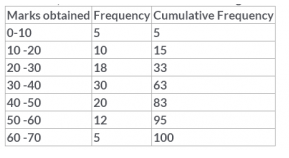Solution:

Median = L + [ (N/2 – F) / f ]C
=30+[(50-33)/30]*10
=35.66
Mode=L+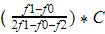=30+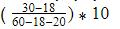=35.45
Mean=(3Median-Mode)/2
=(106.98-35.45)/2=35.76

QUESTION: 3

### If median of 20 observations is 50 and mode is also 50, then the mean is

Solution:
QUESTION: 4

For a symmetrical distribution, which is correct

Solution:

A distribution is symmetrical if a vertical line can be drawn at some point in the histogram such that the shape to the left and the right of the vertical line are mirror images of each other. The mean, the median, and the mode are each equal for these type of data. In a perfectly symmetrical distribution, the mean and the median are the same. In a symmetrical distribution that has two modes (bimodal), the two modes would be different from the mean and median.

QUESTION: 5

The median of the given data is 46 and the total number of items is 230.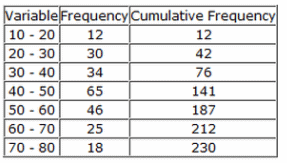Using the formula Mode = 3 Median – 2 Mean, the mean will be

Solution:

To find Mode
Mode=L+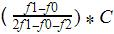Where L=lower limit of the modal class  = 40
f1 = modal class frequency.= 65
f2 = just after the modal class frequency.=46
f0 = just previous the modal class frequency=34
C = Class size=10
Mode =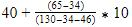=46.2
Mode = 3 Median – 2 Mean
46.2 =3*46-2Mean
2Mean=138-46.2=91.8
Mean=91.8/2=45.9

QUESTION: 6

For the following frequency distribution: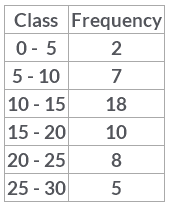If the mode and the median are 12.9 and 14.44 respectively, then the mean is​

Solution:
QUESTION: 7

A student noted the number of cars passing through a spot on a road for 100 periods each of 3 minutes and summarized it in the table given below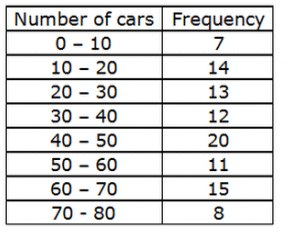The mode, median and mean of the data is​

Solution:
QUESTION: 8

If the median of the following data is 166.79, then the mean and mode are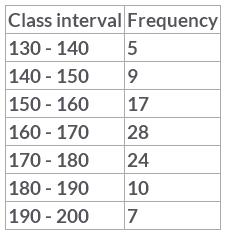Solution:

We have
Mode=L+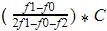Where L=lower limit of the modal class =160
f1= modal class frequency.=28
f2 = just after the modal class frequency.=24
f0 = just previous the modal class frequency=17
C = Class size=10
Mode=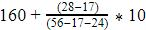=167.3
Mode = 3 Median – 2 Mean
Mean=(3*166.79-167.3)/2=168.03

QUESTION: 9

The mean and the median of a distribution are 45.9 and 46 respectively. The mode will be

Solution:
QUESTION: 10

Which of the following is not a measure of central tendency?

Solution:

A measure of central tendency is a measure that represents the center point or typical value of a data. These measures indicate where most values in a distribution fall and are also referred to as the central location of a distribution.It can also be viewed as the tendency of data to cluster around a middle value.Standard deviation as the name suggests is a measure of the deviation. Deviation means change or distance. But change is always followed by the word 'from'. Hence standard deviation is a measure of change or the distance from a measure of central tendency  which is normally the mean. Hence, standard deviation is different from a measure of central tendency.